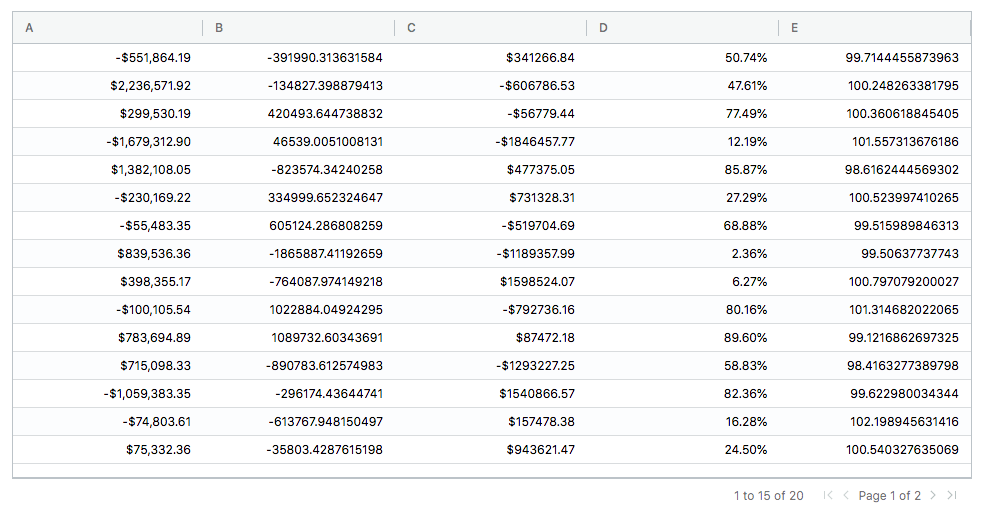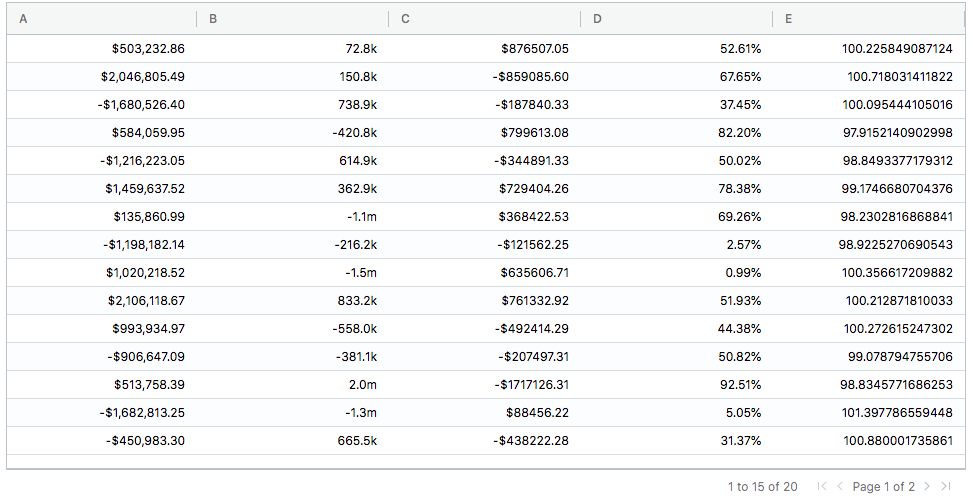# Table Formatting

## Format Columns

Here’s an example of how you can format the table columns as currency , percentages or any options supported by numeraljs.

Please check out numeraljs for all the options that can be used.

``````library(RagGrid)
m = cbind(matrix(rnorm(60, 1e5, 1e6), 20), runif(20), rnorm(20, 100))
# Format Column A,C as currency and Column D as percentage.
formattingOptions <- list(A='\$0,0.00',C='\$00.00',D ='0.00%')
aggrid(m,formattingOptions = formattingOptions)``````Here’s another example for number formatting.Let’s do a million/thousand formatting on Column B. This can be achieved with a format string like ‘0a’

``````library(RagGrid)
m = cbind(matrix(rnorm(60, 1e5, 1e6), 20), runif(20), rnorm(20, 100))